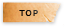# 機率論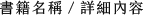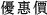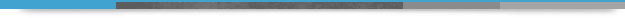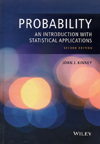## Probability: An Introduction with Statistical Applications 2/e

+作者：

### Kinney

+年份：
2015 年2 版
+ISBN：
9781118947081
+書號：
PS0439H
+規格：

+頁數：
482
+出版商：
John Wiley

\$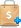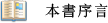"This is a well-written and impressively presented introduction to probability and statistics. The text throughout is highly readable, and the author makes liberal use of graphs and diagrams to clarify the theory."  - The Statistician

Thoroughly updated, Probability: An Introduction with Statistical Applications, Second Edition features a comprehensive exploration of statistical data analysis as an application of probability. The new edition provides an introduction to statistics with accessible coverage of reliability, acceptance sampling, confidence intervals, hypothesis testing, and simple linear regression. Encouraging readers to develop a deeper intuitive understanding of probability, the author presents illustrative geometrical presentations and arguments without the need for rigorous mathematical proofs.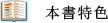• Over 880 problems at varying degrees of difficulty allowing readers to take on more challenging problems as their skill levels increase
• Chapter-by-chapter projects that aid in the visualization of probability distributions
• New coverage of statistical quality control and quality production
• An appendix dedicated to the use of Mathematica® and a companion website containing the referenced data sets

Featuring a practical and real-world approach, this textbook is ideal for a first course in probability for students majoring in statistics, engineering, business, psychology, operations research, and mathematics. Probability: An Introduction with Statistical Applications, Second Edition is also an excellent reference for researchers and professionals in any discipline who need to make decisions based on data as well as readers interested in learning how to accomplish effective decision making from data.
•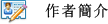John J. Kinney, PhD, is Professor Emeritus of Mathematics at Rose-Hulman Institute of Technology in Terre Haute, Indiana. A Member of the American Statistical Association and the Colorado Council of Teachers of Mathematics, Dr. Kinney is the author of numerous journal articles and three books, including A Probability and Statistics Companion, also published by Wiley.1. Sample Spaces and Probability
2. Discrete Random Variables and Probability Distributions
3. Continuous Random Variables and Probability Distributions
4. Functions of Random Variables; Generating Functions; Statistical Applications
5. Bivariate Probability Distributions
6. Recursions and Markov Chains
7. Some Challenging Problems
Appendix A. Use of Mathematica in Probability and Statistics
Appendix B. Answers for Odd-Numbered Exercises
Appendix C. Standard Normal Distribution